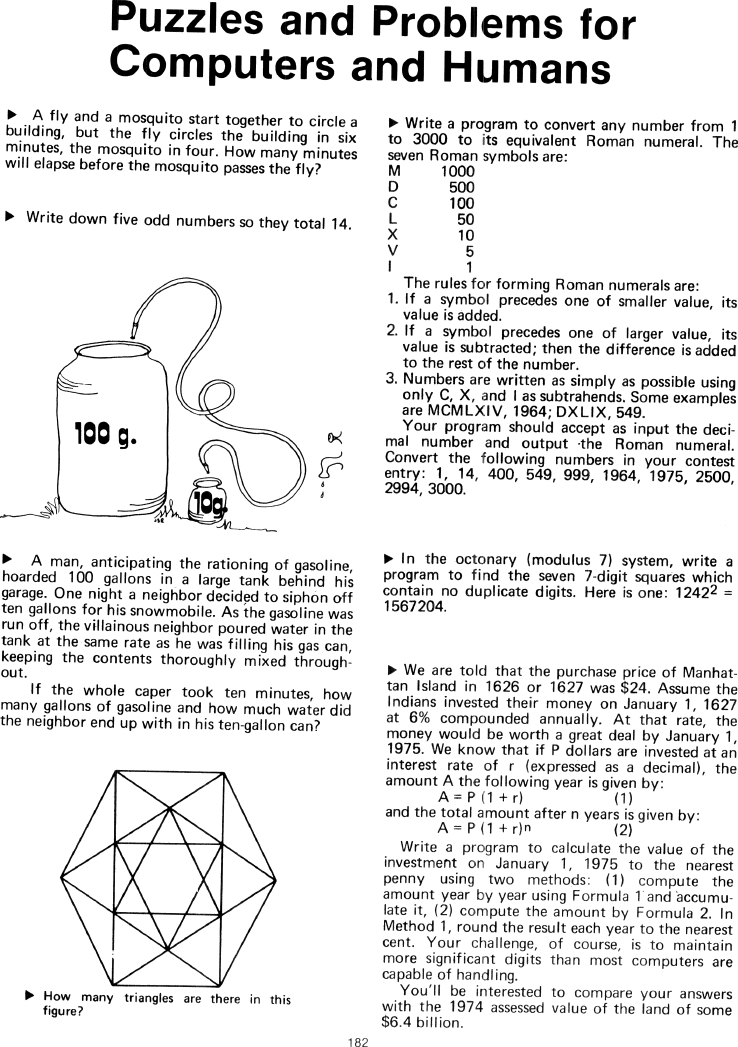The Best of Creative Computing Volume 1 (published 1976)

 `Page 182 << PREVIOUS >> NEXT Jump to page: Go to contents Go to thumbnails`

Puzzles and Problems for Computers and Humans (math puzzles)```Puzzles and Problems for Computers and Humans

> A fly and a mosquito start together to circle a
building, but the fly circles the building in six
minutes, the mosquito in four. How many minutes
will elapse before the mosquito passes the fly?

> Write down five odd numbers so they total 14.

> A man, anticipating the rationing of gasoline,
hoarded 100 gallons in a large tank behind his
garage. One night a neighbor decided to siphon off
ten gallons for his snowmobile. As the gasoline was
run off, the villainous neighbor poured water in the
tank at the same rate as he was filling his gas can,
keeping the contents thoroughly mixed throughout.

If the whole caper took ten minutes, how
many gallons of gasoline and how much water did
the neighbor end up with in his ten-gallon can?

> How many triangles are there in this
figure?

> Write a program to convert any number from 1
to 3000 to its equivalent Roman numeral. The
seven Roman symbols are:

M 1000
D 500
C 100
L 50
X 10
V 5
I 1

The rules for forming Roman numerals are:

1. If a symbol precedes one of smaller value, its value is added.

2. If a symbol precedes one of larger value, its value is subtracted; then the
to the rest of the number.

3. Numbers are written as simply as possible using only C, X, and I as
subtrahends. Some examples
are MCMLXIV, 1964; DXLIX, 549.

Your program should accept as input the decimal number and output the Roman
numeral.

Convert the following numbers in your contest entry: 1, 14, 400, 549, 999, 1964,
1975, 2500,
2994, 3000.

> In the octonary (modulus 7) system, write a
program to find the seven 7-digit squares which
contain no duplicate digits. Here is one: 12422 =
1567204

> We are told that the purchase price of Manhattan Island in 1626 or 1627 was
\$24. Assume the
Indians invested their money on January 1, 1627
at 6% compounded annually. At that rate, the
money would be worth a great deal by January 1,
1975. We know that if P dollars are invested at an
interest rate of r (expressed as a decimal), the
amount A the following year is given by:

A = P (1 + r) (1)
and the total amount after n years is given by:

A = P (1 + r)n (2)
Write a program to calculate the value of the
investment on January 1, 1975 to the nearest
penny using two methods: (1) compute the
amount year by year using Formula 1 and accumulate it, (2) compute the amount by
Formula 2. In
Method 1, round the result each year to the nearest
cent. Your challenge, of course, is to maintain
more significant digits than most computers are
capable of handling.

with the 1974 assessed value of the land of some
\$6.4 billion.Page 182        << PREVIOUS        >> NEXT        Jump to page:                Go to contents        Go to thumbnails

<!--